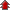# Three Dimensional Geometry

• NCERT Chapter### Exercise 1

•  Q1 If a line makes angles 90°, 135°, 45° with x, y and z-axes respectively, find its direction cosines. Q2 Find the direction cosines of a line which makes equal angles with the coordinate axes. Q3 If a line has the direction ratios −18, 12, −4, then what are its direction cosines? Q4 Show that the points (2, 3, 4), (−1, −2, 1), (5, 8, 7) are collinear. Q5 The vertices of ΔABC are A (3, 5, −4), B (−1, 1, 2), and C (−5, −5, −2).